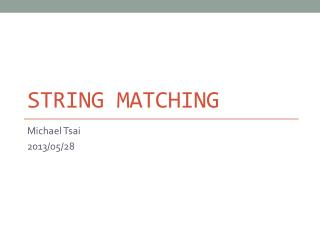DownloadDownload PresentationString Matching

# String Matching

Télécharger la présentation## String Matching

- - - - - - - - - - - - - - - - - - - - - - - - - - - E N D - - - - - - - - - - - - - - - - - - - - - - - - - - -
##### Presentation Transcript

1. String Matching Michael Tsai 2013/05/28

2. 問題: 字串比對 • 陣列T[1..n]中有一個長度為n的字串 • 陣列P[1..m]中有一個長度為m的字串 • 要在T中找P是否出現 • P和T的字串從一個字元的集合中拿出 • 如: 或 • Pattern P occurs withshifts in text T(Pattern P occurs beginning at position s+1 in text T) if , for . • If P occurs with shift s in T, we call s a valid shift. Otherwise, we call s an invalid shift. • 字串比對問題是要在T中間找到所有P出現的位置(valid shift)

3. 一些定義 • : 所有使用中字元組成的有限長度字串(包括長度為0的空字串) • : 字串x的長度 • xy: 把字串x和y接起來 (concatenation) • : 字串w是字串x的prefix (也就是x=wy, ) ( 表示 ) • : 字串w是字串x的suffix (也就是x=yw, ) ( 表示 ) • 例如: ababcca, cca abcca • 空字串為任何字串的prefix & suffix • 對任何字串x, y和字元a, iff • 和為transitive(具遞移律)的operator

4. 方法一: 笨蛋暴力法 Native-String-Matcher(T, P) N=T.length M=P.length for s=0 to n-m if P[1..m]==T[s+1..s+m] print “Pattern occurs with shift” s n-m+1次

5. 為什麼不好? • 因為每次for執行比對, 如果錯了, 這回合的資訊完全丟掉. • 例:P=aaab • 如果我們發現s=0是validshift (表示T開頭為aaab), • 那麼從之前的結果應該可以知道shift 1, 2, 3 都可以直接跳過, 不需要一一比對. T P

6. 方法二: The Rabin-Karp Algorithm • 假設 • 那麼每個長度為k的字串可以想成是一個k位數的十進位數 • 例如字串”31415”可以想成是十進位數31415 • 把設為代表T[s+1..s+m]的十進位數 • p設為代表P的十進位數 • 那麼iffT[s+1..s+m]=P[1..m], 也就是s是valid shift • 怎麼從P計算p呢?

7. 方法二: The Rabin-Karp Algorithm • 怎麼從P計算p呢? 整個往右移一格 拿掉最左邊那一格 加上最右邊那一格

8. 方法二: The Rabin-Karp Algorithm • 那麼用這個方法要多花少時間呢? (簡易分析版) • 然後用, 算的值 (每次都是constant time, 共n-m次) • 所以總共: preprocessing時間, 比對時間

9. 方法二: The Rabin-Karp Algorithm • 之前的兩個問題: • 如果是general的character set, 怎麼辦? (不再是{0,1,…,9}) 如何解決呢? 假設, . 可以把之前的式子改成 (a) 把整個string看成一個d進位的數. (b)字元在中index當作該字元所代表的的值

10. 方法二: The Rabin-Karp Algorithm • 當m比較大的時候, p和將很難用電腦直接處理 (用long long也存不下) 加一加乘一乘最後總是會overflow • 如何解決? 利用同餘理論. Michael Rabin Richard Karp

11. 同餘理論 (Modular Arithmetic) • 假設a, b都為整數 • : 表示a和b除以n的餘數相等 • 例如: • 更棒的性質: • 則

12. 同餘理論 (Modular Arithmetic) • 則 • 證明: • 表示 • 表示 • 所以 • 兩者餘數相同! • 得證.

13. 同餘理論 (Modular Arithmetic) • 則 • 證明: • 表示 • 表示 • 所以 • 兩者餘數相同! 得證!

14. The Rabin-Karp Algorithm 修正版 • 取q使得dq可以用一個電腦word (32-bit or 64-bit)來表示 • 既然mod後再加, 減, 乘也會保持原本的關係, 我們可以把這些operation都變成mod版本的

15. The Rabin-Karp Algorithm 修正版 • 新的mod版algorithm會造成一個問題: • 雖然 • 但 • 例如, • 但是如果 • 所以演算法變成這樣: • 如果, 那麼現在這個s為invalid shift • 如果, 那麼必須額外檢查 (直接比對範圍內的字串花很多時間) • 當, 但是時, 稱為spurious hit • 當q夠大的時候, 希望spurious hit會相當少

16. 例子: Rabin-Karp Algorithm

17. Pseudo Code: Rabin-Karp Rabin-Karp-Matcher(T,P,d,q) n=T.length m=P.length h= mod q p=0 t=0 for i=1 to m p=(dp+P[i]) mod q t=(dt+T[i]) mod q for s=0 to n-m if p==t if P[1..m]==T[s+1..s+m] print “Pattern occurs with shift” s if s<n-m t=(d(t-T[s+1]h)+T[s+m+1]) mod q T: string to be searched P: pattern to be matched d: size of the character set q: max number Pre-processing: 迴圈跑n-m+1次 Hit的時候比對:

18. Worst-case Running Time Rabin-Karp-Matcher(T,P,d,q) n=T.length m=P.length h= mod q p=0 t=0 for i=1 to m p=(dp+P[i]) mod q t=(dt+T[i]) mod q for s=0 to n-m if p==t if P[1..m]==T[s+1..s+m] print “Pattern occurs with shift” s if s<n-m t=(d(t-T[s+1]h)+T[s+m+1]) mod q Worst case的時候: T=(n個a) P=(m個a) 比對的時間為 O(m(n-m+1)) Pre-processing: 迴圈跑n-m+1次 Hit的時候比對:

19. Average Running Time 平常的時候, valid shift很少(假設有c個)不會每次都有modulo的hit. 假設字串各種排列組合出現的機率相等，則spurious hit的機率可當成. Rabin-Karp-Matcher(T,P,d,q) n=T.length m=P.length h= mod q p=0 t=0 for i=1 to m p=(dp+P[i]) mod q t=(dt+T[i]) mod q for s=0 to n-m if p==t if P[1..m]==T[s+1..s+m] print “Pattern occurs with shift” s if s<n-m t=(d(t-T[s+1]h)+T[s+m+1]) mod q Pre-processing: 則比對花的時間: Spurious hit共花O( (n-m+1)/q)=O(n/q)次 總共比對花的時間為 O((n-m+1)+(m(c+n/q))) 迴圈跑n-m+1次 If c=O(1) and qm,  O(n+m)=O(n) Hit的時候比對:

20. 方法三: The Knuth-Morris-Pratt Algo. 一樣耶! 表示等一下可以從P第4個位置開始比 T不用倒退! 時間複雜度會降低!

21. Knuth-Morris-Pratt Don Knuth Vaughan Pratt James Morris

22. 正式一點的說法 • 假設P[1..q]和T[s+1..s+q]已經match了 • 要找出最小的shift s’使得某個k<q可以滿足 If 的suffix s’=s+(q-k) 找的最長prefix使得它是的suffix

23. 最好的狀況下: 沒有重複的pattern s q s’=s+q k=0

24. 先處理P來取得”重複pattern”的資訊 找的最長prefix使得它是的suffix • 定義Prefix function (failure function) : • Input: {1,2,…,m} • Output: {0,1,…,m-1} • (也就是前面例子中的k值, 可以想成最長的重複pattern的長度) 找的最長prefix 使得它是的suffix

25. Prefix function example

26. Pseudo-code: Prefix function Compute-Prefix-Function(P) m=P.length let be a new array k=0 for q=2 to m while k>0 and P[k+1]!=P[q] k= if P[k+1]==P[q] k=k+1 return

27. 例子: Matching • T=BACBABABAABCBAB • 實際的Matching Pseudo Code和計算prefix function非常像 • 請見Cormen p. 1005 KMP-Matcher

28. 算Prefix function花多少時間? Compute-Prefix-Function(P) m=P.length let be a new array k=0 for q=2 to m while k>0 and P[k+1]!=P[q] k= if P[k+1]==P[q] k=k+1 return 麻煩的是這邊: 總共會跳多少次呢?

29. 算Prefix function花多少時間? Compute-Prefix-Function(P) m=P.length let be a new array k=0 for q=2 to m while k>0 and P[k+1]!=P[q] k= if P[k+1]==P[q] k=k+1 return 進入迴圈的時候k<q, 且q每次增加, k有時候不增加 所以k<q永遠成立 所以. 所以每執行一次迴圈就減少k一次且k永遠不是負的 Total:O(m) k只會在這邊增加, 因此最多總共增加m-1次(迴圈執行次數) 最後: 既然有增加才有得減少, while loop總共執行的次數不會超過O(m)

30. KMP執行時間 • 類似的方法可以證明比對的部分執行時間為O(n) • 所以總和來看: • Preprocessing時間O(m) • 比對時間 O(n)

31. Today’s Reading Assignment • Cormench. 32, 32.1, 32.2, 32.4 (正確性的證明略為複雜)